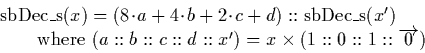Next: Signed decimal fractions to Up: Signed Binary to Decimal Previous: Signed binary integers to

### Signed binary fractions to signed decimal fractions

Conversion to signed decimal is performed as follows. Given the signed binary input u, we compute the decimal resultas:

We implement this using a signed binary stream, representing a number in the interval [-1,1]. This means we cannot represent the value ten to perform multiplication by ten using the signed binary stream multiplication algorithm. It is possible, however, to multiply instead by the value 10/24, which is in the interval [-1,1] and can be represented as a signed binary stream. This produces the correct result provided we interpret the output as being multiplied by 24.

The algorithm for converting from signed binary into signed decimal is therefore as follows:In fact the output stream is in fact not true signed decimal, because we can in fact get the digits -10 and 10 appearing at the first digit if there is an overflow. However this is not a problem because we can easily check these digits in the first output digit when the conversion is complete, and handle the overflow appropriately if necessary.Next: Signed decimal fractions to Up: Signed Binary to Decimal Previous: Signed binary integers to
Martin Escardo
5/11/2000